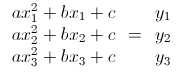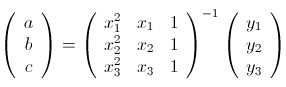# Ruby Matrix, the Forgotten Library

## Ruby Matrix, the Forgotten Library

This guest post is contributed by Matthew Kirk, who is a partner at Modulus 7, specializing in software development and strategy. The basis of his career has been around utilizing science to improve businesses. He has spoken at technology conferences around the world and in his spare time, he enjoys traveling and adding to his 2000+ vinyl record collection.Remember matrices from math class? No not the movie, but the rectangular array of numbers. While you might not see it often, Ruby has a matrix implementation that is well tested and allows you to accomplish tough calculations quickly.

While I won’t be able to teach you everything there is to be known about matrices, we will cover how to use matrices within Ruby as well as some quirks and their major selling points. By the end of this I hope that you delve deeper into learning about matrices and use them in your next project.

### What are matrices?

A matrix according to Wikipedia is a rectangular array of numbers. Used heavily in math, matrices are all over languages like R and Matlab. They can be a great way to store numerical data and simplify many difficult and tedious problems. Instead of solving systems of equations matrices can simplify these into one equation.

In terms of how Ruby implements matrices, Ruby stores all matrix rows into one big array. The only requirement is that the arrays are of the same dimension. So for each row that is added to a matrix each one must be of the same size.

Just like arrays, matrices are zero indexed meaning that the first row is index 0 and the first column is index 0. Unlike arrays though you have to have two indexes to get to an element:

#### Some quirks:

The `Matrix` library has some quirks. The `Matrix` class allows non-numerical data to go into itself. This could be useful for storing things like symbols in a more x, y format but render most of the matrix functions useless.

For instance:

Another quirk to be aware of: `Matrix[*rows]` does not copy the rows objects but instead points to it. To avoid this use `Matrix.rows(rows)` or `Matrix.columns(columns)`. Implementation wise `Matrix[*rows]` calls the function `Matrix.rows(rows, copy = false)`.

#### Iterating over matrices:

How do you iterate over a matrix? Most likely you would think that matrices read left to right top to bottom. And that’s true. But there are other cases as well.

In total there are 7 ways to iterate over a Matrix in ruby:

• `:all` This reads left to right top to bottom. This is the default case when you type `matrix.each`
• `:diagonal`: This only reads the diagonal elements or row index == column index
• `:off_diagonal`: This will read everything not on the diagonal or row index != column index
• `:lower`: This reads the lower triangle of the matrix or row index <= column index
• `:strict_lower`: this is more strict and reads only row index < column index
• `:strict_upper`: this is a strict upper triangle and is row index > column index
• `:upper`: row index >= column index

An example:

#### Example: Parabola with matrix:

Imagine you want to fit a curve through three points. If you remember math class you might remember that you can do this by fitting a quadratic. For instance lets say we want a line that goes through (1,2) (3, 5.5) and (6, 6). To solve this we would write the equations:The way to solve this would usually involve lots of algebra and substitutions. While it’s easy to solve this in the case where we already know the numbers it is difficult to come up with a general solution (try it I dare you).

Instead of worrying about solving this using non-matrix algebra we can solve it using matrix algebra. The first step is to rewrite the above system into this form:To make it even easier to solve we would rewrite this as Ax = b. To solve this we would take the inverse of A and then multiply both sides by that. Which would yield:Now that we know this, we can easily solve this using Ruby with the following formula.

While it’s close it won’t be correct unless you use `Rational`. Ruby’s matrix library graciously utilizes functions that preserve precision. You would expect most libraries to convert to floats but ruby does not.

For instance you can change the above function call to:

Whenever possible try to preserve the precision!

#### The general case, fitting an n-power polynomial to n points:

Up above we only fit this curve to 3 points. But what about 4 or 15 points? This would be quite simple to do and would only require a little bit of modification:

### Conclusion:

While you might not use matrices every day, they can be useful to solve problems involving systems of equations. Ruby has a robust `matrix` library that can be useful in finding solutions to these types of problems. Next time you want to fit a curve keep in mind matrices might be the best way to go!

For more information about matrices I recommend reading Wikipedia articles. There are lots of math professors who spend hours updating them tediously. If they are too confusing, think about picking up a book on matrix algebra like Matrix Computations.

Feel free to ask questions and give feedback in the comments section of this post. Thanks!